Courses

# NEET Previous Year Questions: Gravitation- 1

## 22 Questions MCQ Test Physics Class 11 | NEET Previous Year Questions: Gravitation- 1

Description
This mock test of NEET Previous Year Questions: Gravitation- 1 for JEE helps you for every JEE entrance exam. This contains 22 Multiple Choice Questions for JEE NEET Previous Year Questions: Gravitation- 1 (mcq) to study with solutions a complete question bank. The solved questions answers in this NEET Previous Year Questions: Gravitation- 1 quiz give you a good mix of easy questions and tough questions. JEE students definitely take this NEET Previous Year Questions: Gravitation- 1 exercise for a better result in the exam. You can find other NEET Previous Year Questions: Gravitation- 1 extra questions, long questions & short questions for JEE on EduRev as well by searching above.
QUESTION: 1

### If suddenly the gravitational force of attraction between earth and a satellite revolving around it becomes zero, then the satellite will   [AIEEE 2002]

Solution:

When the gravitational force becomes zero that means the centripetal force required cannot be provided therefore the satellite will tend to move tangential to the original orbit with the same velocity (that the satellite had at the instant before the gravitation force become 0).

QUESTION: 2

### The escape velocity of a body depends upon mass as [AIEEE 2002]

Solution:

We know that escape velocity,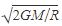Where M is the mass of the planet and R is the radius of the planet.
Thus we can see that v does not depend upon the mass of the object.

QUESTION: 3

### The kinetic energy needed to project a body of mass m from the earth's surface (radius R) to infinity is   [AIEEE 2002]

Solution:

If any object through with escaping velocity then the object will never come back at the ground, So we can assume it will reach at infinite distance.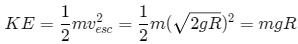QUESTION: 4

Energy required to move a body of mass m from an orbit of radius 2R to 3R is

[AIEEE 2002]

Solution:
QUESTION: 5

The escape velocity for a body projected vertically upwards from the surface of earth is 11 km/s. If the body is projected at an angle of 45° with the vertical, the escape velocity will be

[AIEEE 2003]

Solution:

The escape velocity of a body does not depend on the angle of projection from earth. It is 11 km/s.
Hence C is correct.

QUESTION: 6

Two spherical bodies of mass M and 5M and radii R and 2R respectively are released in free space with initial separation between their centres equal to 12R. If they attract each other due to gravitational force only. then the distance covered by the smaller body just before collision is

[AIEEE 2003]

Solution:

Let the spheres collide after time t1 when the smaller sphere covered distance x1 and bigger sphere covered distance x2.
The gravitational force acting between two spheres depends on the distance which is a variable quantity.
The gravitational force,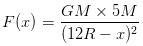Acceleration of smaller body,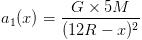Acceleration of bigger body,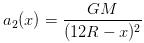From equation of motion,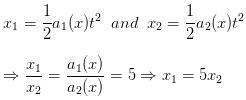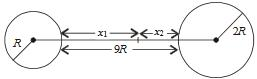We know that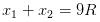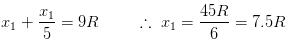Therefore the two spheres collide when the smaller sphere covered the distance of  7.5 R

QUESTION: 7

The time period of a satellite of earth is 5 h. If the separation between the earth and the satellite is increased to 4 times the previous value, the new time period will become

[AIEEE 2003]

Solution: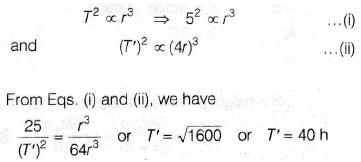QUESTION: 8

Suppose the gravitational forces varies inversely as the nth power of distance. Then the time period of a planet in circular orbit of radius R around the sun will be proportional to

[AIEEE 2004]

Solution:
QUESTION: 9

If g is the acceleration due to gravity on the earth's surface, the gain in the potential energy of an object of mass m raised from the surface of the earth to a height equal to the radius R of the earth, is

[AIEEE 2004]

Solution: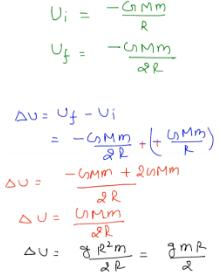QUESTION: 10

The time period of an earth satellite in circular orbit is independent of

[AIEEE 2004]

Solution:

T = 2π a/v
v = velocity of satellite
It is independent of mass of satellite

QUESTION: 11

A satellite of mass m revolves around the earth of radius R at a height x from its surface. If g is the acceleration due to gravity on the surface of the earth, the orbital speed of the satellite is

[AIEEE 2004]

Solution:

½ mv2 = GMm/2r
r = R + x
g = GM/R2
v = [gR2/R+x]2

QUESTION: 12

A particle of mass 10 g is kept on the surface of a uniform sphere of mass 100 kg and radius 10 cm. Find the work to be done against the gravitational force between them, to take the particle far away from the sphere, (you may take G = 6.67 × 10-11 Nm2/kg-2)

[AIEEE 2005]

Solution:

ΔW = Workdone = vf − ui
= 0 − [− GMmR]
ΔW = 6.67 × 10−11× 100 / 0.1 x 10/1000 = 6.67×10−10J

QUESTION: 13

The change in the value of g at a height h above the surface of the earth is the same as at a depth d below the surface of earth. When both and h are much smaller than the radius of earth, then which one of the following is correct ?

[AIEEE 2005]

Solution:
QUESTION: 14

Average density of the earth

[AIEEE 2005]

Solution:
QUESTION: 15

If gE and gM are the accelerations due to gravity on the surfaces of the earth and the moon respectively and if Millikan's oil drop experiment could be performed on the two surfaces, one will find the ratio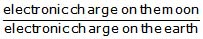to be

[AIEEE 2007]

Solution:

Since electronic charge (1.6 × 10–19C) is a universal constant. It does not depend on g. Therefore, electronic charge on the moon = electronic charge on the earth
Or
(electronic charge on the moon)/ (electronic charge on the earth) = 1

QUESTION: 16

Statement I : For a mass M kept at the centre of a cube of side a, the flux of gravitational field passing through its sides is 4p GM.

and

Statement II : If the direction of a field due to apoint source is radial and its dependence on the distance r from the source is given as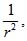its flux through a closed surface depends only on the strength of the source enclosed by the surface and not on the size or shape of the surface.

[AIEEE 2008]

Solution:

Gravity flux:
g × area = 4 π GM
Same as electric flux gravitation flux depends only on the strength of mass hot on shape as in electric flux it depend on the amount of charge not on shape or size of surface.

QUESTION: 17

A planet in a distant solar system is 10 times more massive than the earth and its radius is 10 times smaller. Given that the escape velocity from the earth is 11kms-1, the escape velocity from the surface of the planet would be

[AIEEE 2008]

Solution: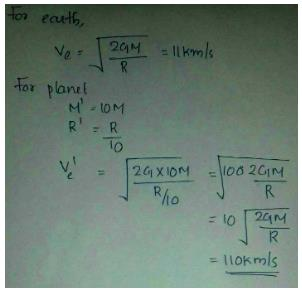QUESTION: 18

The height at which the acceleration due to gravity becomes g/9 (where g = the acceleration due to gravity on the surface of the earth) in terms of R, the radius of the earth is

[AIEEE 2009]

Solution: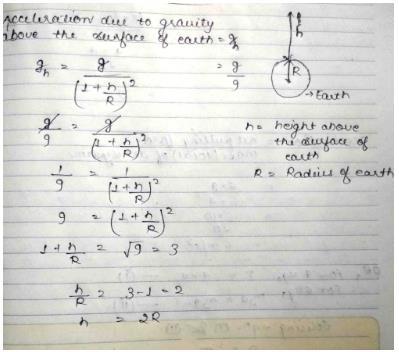QUESTION: 19

Two bodies of masses m and 4 m are placed at a distance r. The gravitational potential at a point on the line joining them where the gravitational field is zero, is

[AIEEE 2011]

Solution:

Let the point be the position when the gravitational field is zero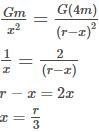the point P is at a distance r/3 from mass m and 2r/3 from mass 4m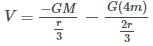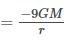QUESTION: 20

Two particles of equal mass m go around a circle of radius R under action of their mutual gravitational attraction. The speed of each particle with respect to their centre of mass is

[AIEEE 2011]

Solution:

Both their masses move in a circular path at exactly opposite diametrically ends with their centripetal forces being the common force of gravitation between them.
I.e. mv2/r = G.m.m/ 4r2
We get v =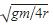QUESTION: 21

The mass of a spaceship is 1000kg. It is to be launched from the earth's surface out into free space. The value of g and R (radius of earth) are 10 m/s2 and 6400 Km respectively. The required energy for this work will be

[AIEEE 2012]

Solution:
QUESTION: 22

What is the minimum energy required to launch a statellite of mass m from the surface of a planet of mass M and radius R in a circular orbit at an altitude of 2R?

[JEE MAIN 2013]

Solution: